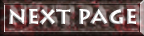# How Base Twelve works

In base ten we arrange our numbers in groups of ten and powers of ten; in base twelve we use groups of twelve and powers of twelve.

In base ten we need to zero (0) and the nine digits from 1 to 9 to count units how many items there are to each power of ten. So the number 15 means "one group of ten and five units";
to write this in base twelve we need to rephrase it as "one group of twelve and three units", i.e. *13. (I use the asterisk, or star, to mark numbers written in base twelve where the context doesn't make it clear.)

So:

Base TenBase Twelve
****
****
**
***
**
****
****
****
***
ten and fivetwelveand three
15*13

## Symbols

If we want to write numbers down then we need number symbols.

The Romans used letters - but the symbols they used, I, V, X, L, C, D and M, were used to record the results of calculations they had made (using pebbles or an abacus), and not to carry out the calculations themselves.

The Roman number XXX records three tens, while III records three units. For the Roman III we write 3 (to stand for three units) and for their XXX we write 30 (to stand for three tens and no units); in our notation the numeral 3 records how many items we are counting, and the position of the numeral tells us which units (tens, hundreds etc) we are counting. Our notation is called positional notation because the position of each numeral is important.

We count in tens; the position of a numeral tells us how many tens or hundreds or higher powers of ten we have, and we say ten is the base of our system. The computer counts in twos and uses powers of two instead of ten.

We write "10" for one ten and no units; so we need only the 0 and nine other symbols (1 to 9) to record all the numbers we may ever want. If we choose a base lower than ten (see the page about number bases and "doggie" for example) then we need less number symbols; and if we choose a base higher than ten, we need more symbols. An example of this is base sixteen, (called "hexadecimal") where we need numerals for the values after nine and before sixteen; for "ten" the symbol "A" has been adopted, "B" for "eleven", up to "F" for "fifteen". The number twenty becomes "14" (sixteen plus four units) in base sixteen, twenty-seven becomes "1B" (sixteen plus eleven units) for example.

I don't like this mixture of letters and numerals, but since base sixteen is only used to record results and translate binary (base two) numbers into a more compact form, I suppose it is not all that important. Using letters will not do, though, if we are considering using a number base other than ten for everyday arithmetic.

Long before the computer reintroduced the topic of number bases into schools the number twelve was being suggested as a better choice for a base for our arithmetic, and in some novels (such as "The Sleeper Awakes" by H.G.Wells) assumed to be the base for the arithmetic of the future...

To count in base twelve we need to add two new symbols,
one for ten and one for eleven,
to the nine (and the zero) we already have
so that "10" may mean "one dozen and no units".

### Onward...

So far, so good. Now -
invent two new symbols......

A simple enough request, yet over the years no-one has come up with two symbols that meet everyone's approval. Before the computer, if you wanted special symbols for your typewriter then they had to be soldered into place. Nowadays we can create symbols with special software - but we haven't quite reached the stage where we can use them in Web documents by embedding the font in which they were created.

I am told this will be possible "soon"; until which time I must type substitutes here for the ones I normally use.

The symbols I and many other dozenists use are the ones suggested by Sir Isaac Pitman (of Pitman Shorthand fame). He proposed and promoted a rotated 2 for ten and a reflected 3 for eleven, their shapes being similar to cursive forms of T and E.

Here are the two symbols: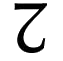for ten, and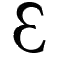for eleven.

Here is the number sequence in base twelve: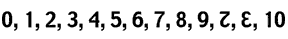(I have used Photoshop to create this line - and later examples - which will do until it becomes possible to embed these fonts I have adapted so that anyone can view the work without actually having the font on his/her computer.)

You can well imagine that there are as many suggestions for the two new symbols as there are people interested in the subject. Some writers would replace the entire set with other designs; some add just the two extra ones needed.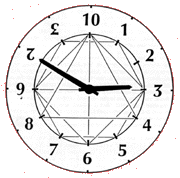Here are the numerals again - and the basic polygons associated with the number twelve - on a clock face:

Twelve divides exactly by 2, 3, 4, 6 and itself - so it is easy to draw the triangle, the square, the hexagon and the duodecagon (with three sides, four, six and twelve) on the clock face simply by joining up the numbers.

 We say that base twelve is better than base ten. One of the reasons for this can be seen in the patterns shown in the multiplication table.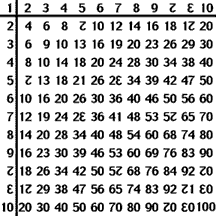The line for multiples of two looks familiar - any number that can be divided exactly by two ends in an even number (or 0);
the line for 3, however, is a lot simpler, and this is because twelve divides exactly by 3 - so any number ending in -0 (a multiple of twelve) is divisible by 3, as is any number ending in other multiples of 3 such as 3, 6 and 9.

A similar argument applies to 4 and 6, and the 6-line corresponds to the 5-line in the base ten multiplication table.

Since *100 (or *10 x *10, twelve squared) divides exactly by 8 and by 9 the lines for 8 and 9 are simplified and the patterns in the multiples are also easy to see.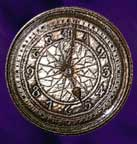But, you may ask - what about 5? 7? and the two new numerals? Neither 5 nor 7 divides twelve exactly, so the patterns are not obvious (nor is that for 7 in base ten); ten being twice five, its pattern is not as obvious. As for eleven, this looks like the 9-line in base ten: in base ten multiples of 9 add to a number divisible by 9 (nine is one less than ten); in the same way, in base twelve (eleven is one less than twelve) multiples of eleven add to numbers divisible by eleven. This also means, by the way, that prime numbers written in base twelve will end in 1, 5, 7 and eleven (but not, of course, that all numbers ending in these digits will be prime).